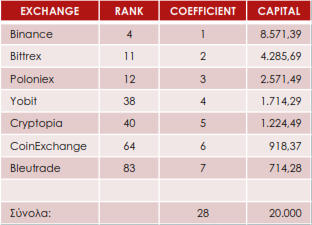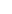The platforms we have selected to make our investments are listed in the first column of the table below. Their ranking according to their trading volume is shown in the second column of the table and in the third column we place the risk degree coefficients, where the platform with the smallest trading volume bears the highest coefficient. The fourth column shows the capital that should be placed on each platform separately and we complete its fields - starting from the end - as follows:

CAP7 = TCAP/TCO = 20.000/28 = 714,28

CAP6 = (TCAP - CAP7)/(TCO – CO7) = (20.000 – 714,28)/(28 – 7) = 19,285,72/21 = 918,37

CAP5 = [TCAP – (CAP7 + CAP6)]/[(TCO – (CO7 + CO6)] = 18367,35/15 = 1224,49 and so on.

Where:

CAPx = Capital on the platform x

TCAP = The total capital we have

COx = The risk degree coefficient of platform x

TCO = The sum of the risk coefficients---to signal pageCapital Allocation: Handling ❺❺❺ - average rating 5 from 5 (based on 201 user reviews)